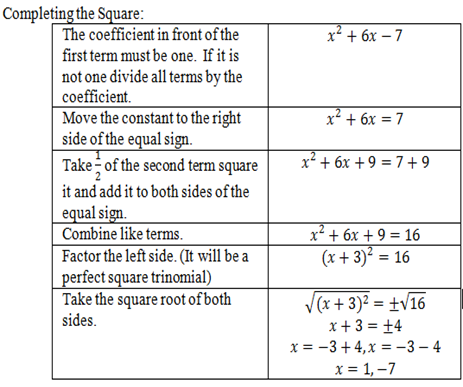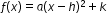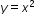# Access Point #: MAFS.912.A-SSE.2.AP.3d

Write expressions in equivalent forms by completing the square to convey the vertex form, to find the maximum or minimum value of a quadratic function, and to explain the meaning of the vertex.
Clarifications:

#### Essential Understandings

Concrete:

• Use a graphing tool or graphing software to find the roots (where the function intersects the x-axis) of a function.
• Use manipulatives (i.e. algebra tiles) to simplify or to write equivalent forms of quadratic functions.
• Use algebra tiles or manipulatives to complete the square.
• Use the graph of the quadratic equation to identify the vertex of the function.
• Identify the maximum or minimum of the graph of the quadratic equation.Representation:
• Understand the following concepts and vocabulary: root, factor, quadratic, integer, real number, quadratic equation, quadratic formula, square root, solution, terms, coefficient, intercept, intersect, zero, completing the square, vertex form, maximum, minimum, vertex, trinomial.
• Use steps or a template to complete the square.• Understand quadratic equations can be rewritten in vertex form.
• Understand that the vertex of a quadratic function is represented by (h, k). The vertex of a quadratic function is the maximum/minimum coordinate of the function. If the function opens up it is a minimum, if the function opens down it is a maximum.

For example: y=3(x+2)2-4 (Vertex form)
Vertex (h, k) = (-2, 4)
The vertex will be moved 2 units to the left and 4 units up from (0,0), the vertex of the parent function.

General Information
Number: MAFS.912.A-SSE.2.AP.3d
Category: Access Points
Cluster: Write expressions in equivalent forms to solve problems. (Algebra 1 - Supporting Cluster) (Algebra 2 - Major Cluster)

Clusters should not be sorted from Major to Supporting and then taught in that order. To do so would strip the coherence of the mathematical ideas and miss the opportunity to enhance the major work of the grade with the supporting clusters.

## Related Standards

This access point is an alternate version of the following benchmark(s).

## Related Courses

This access point is part of these courses.
1200310: Algebra 1
1200320: Algebra 1 Honors
1200330: Algebra 2
1200340: Algebra 2 Honors
1200380: Algebra 1-B
1200400: Foundational Skills in Mathematics 9-12
1207310: Liberal Arts Mathematics
1200410: Mathematics for College Success
1200700: Mathematics for College Algebra
7912060: Access Informal Geometry
7912070: Access Liberal Arts Mathematics
7912080: Access Algebra 1A
7912090: Access Algebra 1B
1200315: Algebra 1 for Credit Recovery
1200335: Algebra 2 for Credit Recovery
1200385: Algebra 1-B for Credit Recovery
7912065: Access Geometry
7912075: Access Algebra 1
7912095: Access Algebra 2
1200387: Mathematics for Data and Financial Literacy

## Related Resources

Vetted resources educators can use to teach the concepts and skills in this access point.

## Student Resources

Vetted resources students can use to learn the concepts and skills in this access point.

## Parent Resources

Vetted resources caregivers can use to help students learn the concepts and skills in this access point.